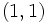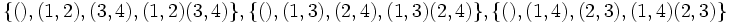# Subgroup structure of symmetric group:S4

View subgroup structure of particular groups | View other specific information about symmetric group:S4

The symmetric group of degree four has many subgroups.

Note that since$S_4$ is a complete group, every automorphism is inner, so the classification of subgroups upto conjugacy is equivalent to the classification of subgroups upto automorphism. In other words, every subgroup is an automorph-conjugate subgroup.

1. The trivial subgroup. Isomorphic to trivial group.(1)
2. The two-element subgroup generated by a transposition, such as$(1,2)$. Isomorphic to cyclic group of order two.(6)
3. The two-element subgroup generated by a double transposition, such as$(1,2)(3,4)$. Isomorphic to cyclic group of order two. (3)
4. The four-element subgroup generated by two disjoint transpositions, such as$\langle (1,2) \ , \ (3,4) \rangle$. Isomorphic to Klein four-group. (3)
5. The unique four-element subgroup comprising the identity and the three double transpositions. Isomorphic to Klein four-group. (1)
6. The four-element subgroup spanned by a 4-cycle. Isomorphic to cyclic group of order four.(3)
7. The eight-element subgroup spanned by a 4-cycle and a transposition that conjugates this cycle to its inverse. Isomorphic to dihedral group of order eight. This is also a 2-Sylow subgroup. (3)
8. The three-element subgroup spanned by a three-cycle. Isomorphic to cyclic group of order three.(4)
9. The six-element subgroup comprising all permutations that fix one element. Isomorphic to symmetric group on three elements. (4)
10. The alternating group: the subgroup of all even permutations. Isomorphic to alternating group:A4.(1)
11. The whole group.(1)

## Tables for quick information

### Table classifying subgroups up to automorphisms

Automorphism class of subgroups Isomorphism class Number of conjugacy classes Size of each conjugacy class Isomorphism class of quotient (if exists) Subnormal depth (if subnormal)
trivial subgroup trivial group 1 1 -- 1
S2 in S4 cyclic group:Z2 1 6 -- --
subgroup generated by double transposition in S4 cyclic group:Z2 1 3 -- 2
A3 in S4 cyclic group:Z3 1 4 -- --
Z4 in S4 cyclic group:Z4 1 3 -- --
normal Klein four-subgroup of S4 Klein four-group 1 1 symmetric group:S3 1
non-normal Klein four-subgroups of S4 Klein four-group 1 3 -- --
S3 in S4 symmetric group:S3 1 4 -- --
D8 in S4 dihedral group:D8 1 3 -- --
A4 in S4 alternating group:A4 1 1 cyclic group:Z2 1
whole roup symmetric group:S4 1 1 trivial group 0

### Table classifying isomorphism types of subgroups

Group name GAP ID Occurrences as subgroup Conjugacy classes of occurrence as subgroup Occurrences as normal subgroup Occurrences as characteristic subgroup
Trivial group$(1,1)$ 1 1 1 1
Cyclic group:Z2$(2,1)$ 9 2 0 0
Cyclic group:Z3$(3,1)$ 4 1 0 0
Cyclic group:Z4$(4,1)$ 3 1 0 0
Klein four-group$(4,2)$ 4 2 1 1
Symmetric group:S3$(6,1)$ 4 1 0 0
Dihedral group:D8$(8,3)$ 3 1 0 0
Alternating group:A4$(12,3)$ 1 1 1 1
Symmetric group:S4$(24,12)$ 1 1 1 1
Total -- 30 11 4 4

### Table listing number of subgroups by order

Note that these orders satisfy the congruence condition on number of subgroups of given prime power order: the number of subgroups of order$p^r$ is congruent to$1$ modulo$p$.

Group order Occurrences as subgroup Conjugacy classes of occurrence as subgroup Occurrences as normal subgroup Occurrences as characteristic subgroup
1 1 1 1 1
2 9 2 0 0
3 4 1 0 0
4 7 3 1 1
6 4 1 0 0
8 3 1 0 0
12 1 1 1 1
24 1 1 1 1
Total 30 11 4 4

### Table listing numbers of subgroups by group property

Group property Occurrences as subgroup Conjugacy classes of occurrence as subgroup Occurrences as normal subgroup Occurrences as characteristic subgroup
Cyclic group 17 5 1 1
Abelian group 21 7 2 2
Nilpotent group 24 8 2 2
Solvable group 30 11 5 5

### Table listing numbers of subgroups by subgroup property

Subgroup property Occurences as subgroup Conjugacy classes of occurrences as subgroup Automorphism classes of occurrences as subgroup
Subgroup 30 11 11
Normal subgroup 5 5 5
Characteristic subgroup 5 5 5
Fully characteristic subgroup 5 5 5
Self-centralizing subgroup 20 8 8
Pronormal subgroup 21 8 8
Retract 12 4 4

## The alternating group (twelve-element characteristic subgroup) (type (10))

This is a characteristic subgroup. The alternating group inside the symmetric group on the set$\{ 1,2,3,4 \}$ is given as:$\{ (), (1,2,3), (1,3,2), (1,2,4), (1,4,2), (2,3,4), (2,4,3), (1,3,4), (1,4,3), (1,3)(2,4), (1,4)(2,3), (1,2)(3,4) \}$

### Subgroup-defining functions yielding this subgroup

• The commutator subgroup.
• The Jacobson radical: It is, in fact, the unique maximal normal subgroup.
• The subgroup generated by squares.
• The Sylow-closure for the prime$3$.

### Subgroup properties satisfied by this subgroup

The alternating group is a verbal subgroup on account of being generated by commutators, or equivalently, on account of being generated by squares (actually, the two facts are closely related, and have to do with the fact that the symmetric group is a group generated by involutions). Thus, it satisfies some subgroup properties, including:

It also satisfies some other subgroup properties, such as:

## The Klein four-group of double transpositions (type (5))

This is a characteristic subgroup. In the symmetric group on the set$\{ 1,2,3,4\}$, it is given as:$\{ (), (1,2)(3,4), (1,3)(2,4), (1,4)(2,3) \}$

### Subgroup properties satisfied by this subgroup

The given subgroup is a verbal subgroup on account of being the second member of the derived series. Thus, it satisfies some subgroup properties, including:

It also satisfies some other subgroup properties, such as:

### Subgroup properties not satisfied by this subgroup

This subgroup can be obtained as follows. Consider the Klein four-group, and use the embedding of this group inside the symmetric group on four letters given by Cayley's theorem. The image is precisely this subgroup.

## The two-element subgroup generated by a transposition (type (2))

There are six such subgroups. In the symmetric group on$\{ 1,2,3,4\}$, these subgroups are given by:$\{ (), (1,2) \}, \{ (), (2,3) \}, \{ (), (1,3) \}, \{ (), (1,4), \}, \{ (), (2,4) \} \{ (), (3,4) \}$

### Effect of operators

• Centralizer: The centralizer is a Klein four-group, of type (4).
• Normalizer: The normalizer is a dihedral group of order eight, of type (7).

## The two-element subgroup generated by a double transposition (type (3))

There are three such subgroups. In the symmetric group on$\{ 1,2,3,4\}$, these are given by:$\{ (), (1,2)(3,4) \}, \{ (1,3)(2,4) \}, \{ (1,4)(2,3) \}$.

These three subgroups are in bijection with the three 2-Sylow subgroups of order eight (type (7)) via the normalizer operation.

### Effect of operators

• Normal closure: The normal closure is the Klein four-group, type (5).
• Normalizer: The normalizer is a dihedral group. Each of these subgroups has a different dihedral group as its normalizer.
• Centralizer: The centralizer is the same as the normalizer.

## The non-normal Klein four-groups (type (4))

There are three such groups. These three groups are in bijection with the three 2-Sylow subgroups, via the normalizer operation.$\{ (), (1,2), (3,4), (1,2)(3,4) \}, \{ (), (1,3), (2,4), (1,3)(2,4) \}, \{ (), (1,4), (2,3), (1,4)(2,3) \}$

### Effect of operators

• Normal closure: The normal closure of any of these is the whole group.
• Normalizer: The normalizer of any of these is a dihedral group of order eight (type (7)). This establishes a bijection between the subgroups of type (4) and the subgroups of type (7).
• Centralizer: The centralizer equals the subgroup itself.

## Cyclic subgroup of order four (type (6))

There are three such subgroups, given as follows:$\{ (), (1,2,3,4), (1,3)(2,4), (1,4,3,2) \}, \{ (), (1,2,4,3), (1,4)(3,2), (1,3,4,2) \}, \{ (), (1,3,2,4), (1,2)(3,4), (1,4,2,3)\}$.

### Effect of operators

• Normalizer: The normalizer of any cyclic subgroup is a dihedral group of order eight. In fact, this establishes a bijection between the three cyclic subgroups of order four (type (6)) and the three dihedral subgroups of order eight (type (7)).
• Centralizer: Each subgroup is its own centralizer.
• Normal closure: The normal closure of each such subgroup is the whole group.

This subgroup of the symmetric group on four elements can be obtained by starting with an abstract cyclic group of order four, and then using Cayley's theorem to embed it in the symmetric group on four letters.

## Eight-element subgroups (type (7))

There are three of these subgroups:$\{ (1,2,3,4), (1,3)(2,4), (1,4,3,2), (), (1,3), (2,4), (1,2)(3,4), (1,4)(3,2) \}$$\{ (1,2,4,3), (1,4)(3,2), (1,3,4,2), (), (1,4), (3,2), (1,2)(3,4), (1,3)(2,4) \}$$\{ (1,3,2,4), (1,2)(3,4), (1,4,2,3), (), (1,2), (3,4), (1,3)(2,4), (1,4)(2,3) \}$

### Effect of operators

• Normal closure: The normal closure of any of these is the whole group.
• Normal core: The normal core of any of these is the Klein four-group, type (5).
• Normalizer: Each of these equals its normalizer.
• Centralizer: The centralizer of each of these equals its center, which is generated by the double transposition that is the square of its 4-cycle.

### Relation with other subgroups

• Type (3) (double transposition): There is a bijection between the subgroups generated by double transpositions and the subgroups of order eight, given by either the centralizer or the normalizer operation.
• Type (4) (pair of disjoint transpositions): There is a bijection between the subgroups generated by disjoint pairs of transpositions and the dihedral groups, given by the normalizer operation.
• Type (6) (cyclic of order four): There is a bijection between the subgroups generated by 4-cycles and the subgroups of order eight, given by the normalizer operation.

## The three-element subgroup spanned by a 3-cycle (type (8))

There are four such subgroups:$\{ (), (1,2,3), (1,3,2) \}, \{ (), (1,2,4), (1,4,2) \}, \{ (), (2,3,4), (2,4,3) \}, \{ (), (1,3,4), (1,4,3) \}$

### Effect of operators

• Normalizer: The normalizer is a symmetric group on the three letters moved by the 3-cycle (subgroup of type (9)). This establishes a bijection between the subgroups of types (8) and (9).
• Centralizer: This is the subgroup itself.
• Normal closure: The normal closure is the alternating group (type (10)).
• Normal core: The normal core is trivial.

## The six-element subgroup comprising all permutations that fix one element (type (9))

There are four such subgroups, depending on which element we choose to fix.$\{ (), (1,2,3), (1,3,2), (1,2), (1,3), (2,3) \}, \{ (), (1,2,4), (1,4,2), (1,2), (1,4), (2,4) \}, \{ (), (1,3,4), (1,4,3), (1,3), (1,4), (3,4) \}, \{ (), (2,3,4), (2,4,3), (2,3), (2,4), (3,4) \}$.